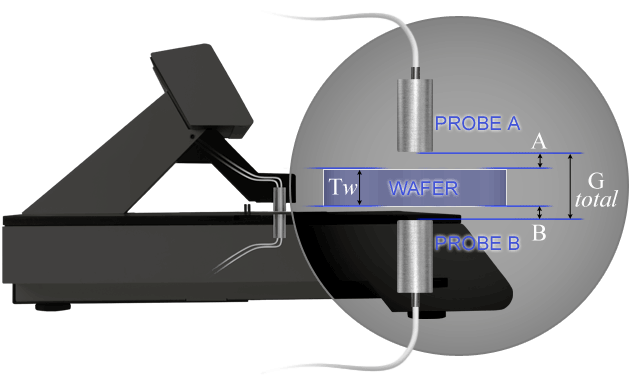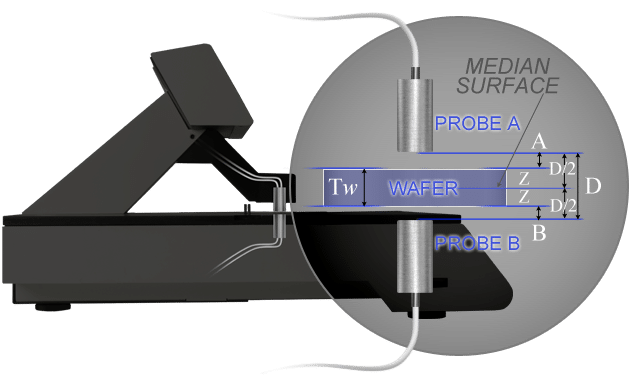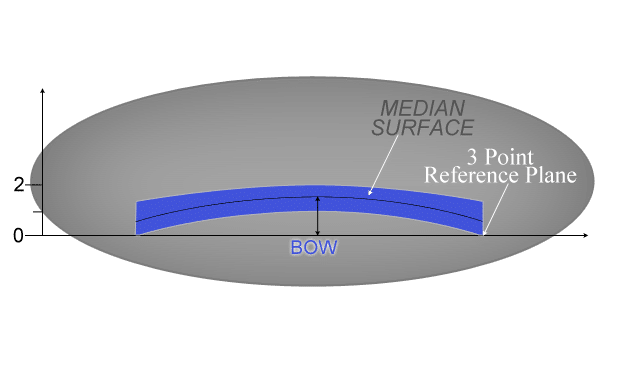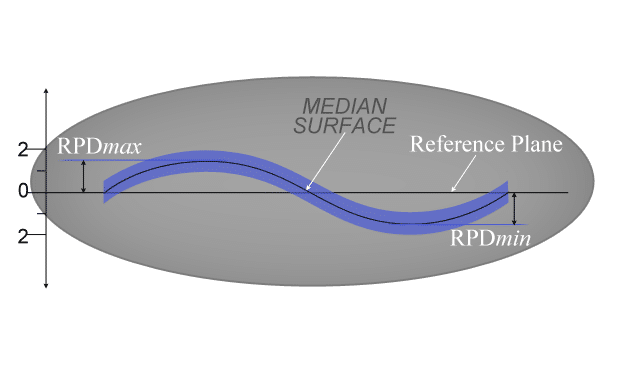# Thickness Measurement

### ASTM F657:

The distance through a wafer between corresponding points on the front and back surface. Thickness is expressed in microns or mils (thousandths of an inch).

# Total Thickness Variation (TTV)

### ASTM F657:

The difference between the maximum and minimum values of thickness encountered during a scan pattern or series of point measurements. TTV is expressed in microns or mils (thousandths of an inch).Figure above shows a wafer placed between two non-contact measurement probes. By monitoring changes between the upper probe face and the upper wafer surface (A) and the bottom probe face and the bottom wafer surface (B), thickness can be calculated. First the system must be calibrated with a wafer on known thickness (Tw). The area of known thickness is placed between the probes and an upper probe to wafer gap (A) and a lower probe to wafer gap (B) is acquired. The total gap (Gtotal) between the upper and lower probes is then calculated as follows:

#### Gtotal = A + B + Tw

With the system calibrated, wafers of unknown thickness can now be measured. When the wafer is placed between the probes, a new value of A and B is acquired. Thickness is calculated as follows:

#### Tw = Gtotal – (A + B)

During an automated scanning of the wafer, a series of point measurements is taken and stored. Following completion of the scan, TTV is calculated as follows:

# BOW MEASUREMENT

### ASTM F534 3.1.2:

The deviation of the center point of the median surface of a free, unclamped wafer from the median surface reference plane established by three points equally spaced on a circle with a diameter a specified amount less than the nominal diameter of the wafer.

## Median Surface:

The locus of points in the wafer equidistant between the front and back surfaces. When measuring and calculating bow, it is important to note that the location median surface of the wafer must be known. By measuring deviations of the median surface, localized thickness variations at the center point of the wafer are removed from the calculation.Above shows the relationship of the wafer median surface between the two probe faces where:

• #### Z = Distance between wafer median surface and the point halfway between the upper and lower probe (D/2)

To determine the value of Z at any location on the wafer, there are two equations:

#### Z = D/2 ‚Äì A ‚Äì T/2 ¬† ¬† ¬† ¬† ¬† and ¬† ¬† ¬† ¬† ¬† Z= -D/2 + B + T/2

Solving both equations for Z, the value can be determined simply by:

#### Z = (B ‚Äì A)/2

Since bow is measured at the center point of the wafer only, a three (3) point reference plane about the edge of the wafer is calculated. The value of bow is then calculated by measuring the location of the median surface at the center of the wafer and determining it‚Äôs distance from the reference plane. Note that bow can be a positive or negative number. Positive denotes the center point of the median surface is above the three point reference plane. Negative denotes the center point of the median surface is below the three point reference plane.# WARP MEASUREMENT

### ASTM F1390:

The differences between the maximum and minimum distances of the median surface of a free, unclamped wafer from a reference place. Like bow, warp is a measurement of the differentiation between the median surface of a wafer and a reference plane. Warp, however, uses the entire median surface of the wafer instead of just the position at the center point. By looking at the entire wafer, warp provides a more useful measurement of true wafer shape. The location of the median surface is calculated exactly as it is for bow and shown above. For warp determination, there are two choices for construction of the reference plane. One is the same three point plane around the edge of the wafer. The other is by performing a least squares fit calculation of median surface data acquired during the measurement scan. Warp is then calculated by finding the maximum deviation from the reference plane (RPDmax) and the minimum differentiation from the reference plane (RPDmin). RPDmax is defined as the largest distance above the reference plane and is a positive number. RPDmin is the largest distance below the reference plane and is a negative number.Figure above is an illustration of the warp calculation. In this example RPDmax is 1.5 and is shown as the maximum distance of the median surface above the reference plane. RPDmin is ‚Äì 1.5 and is shown as the maximum distance of the median surface below the reference plane. Note warp is always a positive value.

#### Warp = 1.5 ‚Äì (-1.5) = 3

It also illustrates the usefulness of taking both bow and warp readings. The median surface of the wafer shown intersects the reference plane at the wafer center, therefore, bow measurement would be zero. The calculated warp value is more useful in this case as it tells the user the wafer does have shape irregularities.